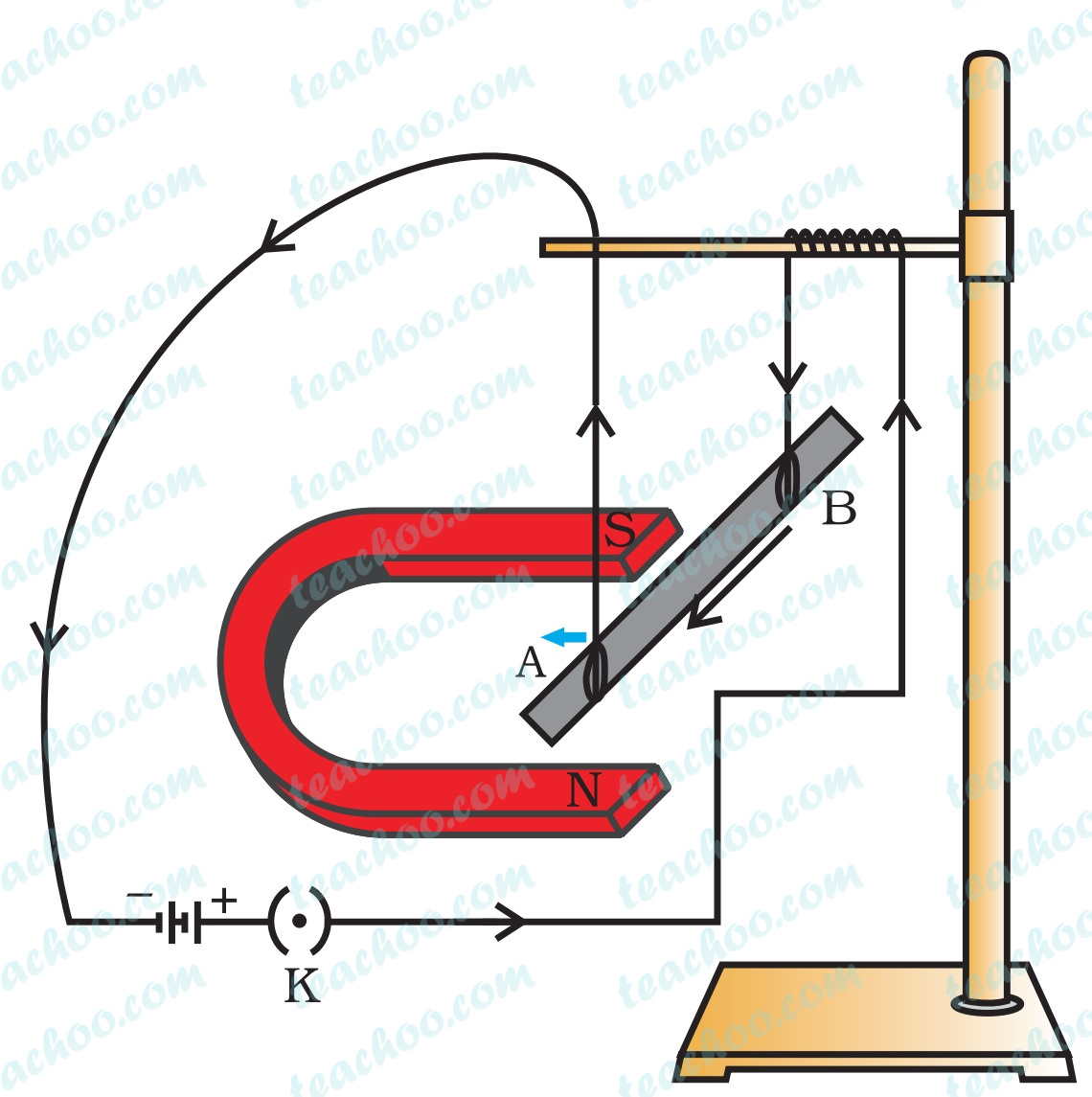Questions from Inside the chapter

Class 10
Chapter 13 Class 10 - Magnetic Effects of Electric Current

## In Activity 13.7, how do we think the displacement of rod AB will be affected if (i) current in rod AB is increased; (ii) a stronger horse-shoe magnet is used; and (iii) length of the rod AB is increased?

We know that

The force by a magnet on a current carrying conductor depends upon

1. The strength of the magnetic field
Magnetic force exerted by a magnet is directly proportional to the strength of magnetic field.

2. The amount of current flowing in the conductor
Magnetic force exerted by a magnet is directly proportional to the amount of current flowing in the conductor.

3. The length of the conductor
Magnetic force exerted by a magnet is directly proportional to the length of the conductorNow, let's solve the question

1. current in rod AB is increased
If current in rod AB will increases, the force will also increase.
Since force increases, displacement will also increase.

2. a stronger horse-shoe magnet is used
If a stronger magnet is used, the strength of magnetic field increases.
Since magnetic field increases, force will also increase
Hence,displacement will also increase

3. length of the rod AB is increased
If length of conductor increases, the force will also increase
Since force increases, displacement will also increase.

Hence, in all 3 cases, displacement will increase

Learn in your speed, with individual attention - Teachoo Maths 1-on-1 Class